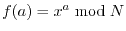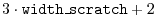Subsections

# Quantum algorithms

## quantum_exp_mod_n

 extern void quantum_exp_mod_n(int N, int x, int width_input, int width_scratch, quantum_reg *reg);

This function uses a quantum algorithm to calculate the function. width_input is the width of the working space, width_scratch is the width of a single scratch space register. Note that the function requires a scratch space ofqubits.

## quantum_qft

 extern void quantum_qft(int width, quantum_reg *reg);

This function performs a Quantum Fourier Transform (QFT). It can be used to determine the period of the values in a quantum register. The QFT is done on the first (i.e. least significant) width qubits of the register.

## quantum_qft_inv

 extern void quantum_qft_inv(int width, quantum_reg *reg);

This is the inverse of the QFT and thus maps the frequency of the basis states into a periodic function.